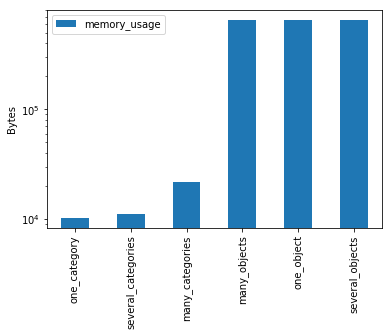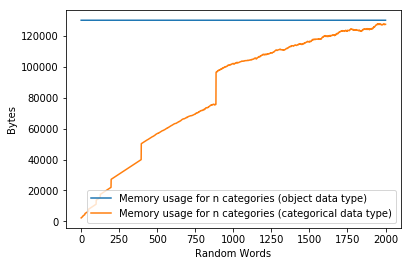# Memory Usage of Pandas Categoricals

A great method to improve memory usage of Pandas DataFrames is by converting columns with categorical variables to use the data type `categorical`. The Pandas documentation explains further how to use this data type in your columns here. In this article we want to explore what memory savings we can typically expect when working with them.

# 1. Setup

First, we import Pandas, NumPy and Matplotlib. Furthermore, we adjust the maximum amount of rows shown when displaying Pandas DataFrames in Jupyter notebooks and the default figure size for Matplotlib.

``````%matplotlib inline
import string

import pandas as pd
import numpy as np
import matplotlib.pyplot as plt

pd.set_option('display.max_rows', 6)

plt.rcParams["figure.figsize"] = 10, 5
``````

# 2. Test Data

We will then create some test data. Since we are concerned with efficiently storing categorical variables, we will create random strings that we then put into a Pandas Series.

First, we define the function that creates `n` random words. Here, we simply choose random letters out of `string.ascii_letters`, which can be found in the Python 3 standard library. We also seed `numpy.random` with `1` just to get consistent results.

``````def random_words(n, word_length=8):
np.random.seed(1)
random_chars = np.random.choice(
list(string.ascii_letters),
(n, word_length),
)
return np.array([
"".join(word)
for i, word in enumerate(random_chars)
])
``````

Let’s take a look at two example random words.

``````random_words(2)
``````

Output:

``````array(['LRmijlfp', 'aqbmhTgz'], dtype='<U8')
``````

We then move on to create the function that gives us a random Pandas Series of words. For this, we invoke the `random_words` function we created before and choose however many different words we need to create a Series of length `length` with `n_categories` different categories.

We also allow specifying a third argument called `categorical`, which will us to decide whether we want the data to be stored as plain Python objects or memory efficient Pandas categoricals. This is important for later benchmarking as we will see.

``````def random_words_series(length, n_categories, categorical=True):
np.random.seed(1)
categories = random_words(n_categories)
words = np.random.choice(
categories,
length,
)
return pd.Series(
words,
dtype='category' if categorical else object,
)
``````

As an example, this is how it would look like to create a series of random words out of 3 different categories with total series length 6.

``````example = random_words_series(
6, 3
)
example.to_frame()
``````

Output:

0
0 aqbmhTgz
1 YuLsulQC
2 YuLsulQC
3 LRmijlfp
4 aqbmhTgz
5 aqbmhTgz

We can then easily see that this Series has exactly 3 categories by inspecting its `.dtype` attribute.

``````len(example.dtype.categories)
``````

Output:

``````3
``````

Now, to compare a few examples, we create Series with 3 different amounts of categories: 1, 10 and 100. Each Series will have a total length of 10000 values. Furthermore, for comparison we create each Series twice: once with the data type set to categorical and once to just using Python objects. We get 6 series in total.

The 3 series ending on `_category` contain data stored as category data in Pandas. The 3 series ending on `_object` contain data stored as Python objects.

``````n = 10000

series = {
'one_category': random_words_series(
n, 1,
),
'several_categories': random_words_series(
n, 10,
),
'many_categories': random_words_series(
n, 100,
),
'one_object': random_words_series(
n, 1, False,
),
'several_objects': random_words_series(
n, 10, False,
),
'many_objects': random_words_series(
n, 100, False,
),
}
``````

After we have created the 6 different series using our `random_words_series` method, we want to move on to analyzing memory usage. This will allow us to find out how big the memory savings are.

# 3. Memory

For each example series, we analyze the memory usage by using the standard Pandas `.memory_usage()` method. We print it out immediately.

``````memory_usage = pd.Series(
{k: v.memory_usage(deep=True) for k, v in series.items()},
name='memory_usage',
dtype='uint64',
).sort_values()
memory_usage.to_frame()
``````

Output:

memory_usage
one_category 10225
several_categories 11050
many_categories 21700
many_objects 650080
one_object 650080
several_objects 650080

We can see that category data in Pandas use considerably less memory than plain Python objects.

Furthermore, we print out a logarithmic plot of memory size for each Series.

``````fig, ax = plt.subplots()
ax.set_ylabel('Bytes')
memory_usage.to_frame().plot(
kind='bar',
logy=True,
ax=ax,
);
``````

Output:Finally, we will try to see how memory usage behaves when the amount of different categories inside a Series grows.

# 4. Memory usage as function of size

For this analysis, we will create 2000 series with up to 2000 different categories. As before, in order to allow us to understand the memory usage of category data in Pandas, we create the series once using the Pandas category data type and once just using plain Python objects.

First, we calculate the memory usage for the plain Python object series:

``````size_n = 2000
size = pd.Series(
{
i: random_words_series(
size_n, i, False,
).memory_usage(deep=True)
for i in range(1, size_n + 1)
},
name="Memory usage for n categories (object data type)",
).sort_values()
``````

Right after that, we calculate the memory usage for all of the categorical series:

``````size_categorical = pd.Series(
{
i: random_words_series(
size_n, i,
).memory_usage(deep=True)
for i in range(1, size_n + 1)
},
name="Memory usage for n categories (categorical data type)",
).sort_values()
``````

Now, we can put the sizes next to each other in a Pandas DataFrame. We will immediately see that despite the number of categories nearing 2000, the memory usage never comes close to that of the Series using plain Python objects.

``````sizes = pd.DataFrame([size, size_categorical]).T
sizes
``````

Output:

Memory usage for n categories (object data type) Memory usage for n categories (categorical data type)
1 130080 2225
2 130080 2290
3 130080 2355
... ... ...
1998 130080 127460
1999 130080 127395
2000 130080 127460

We can plot these values against each other. Since the Python object Series always contain the same amount of data regardless of the number of categories we see that the memory usage is constant as well. For the category data type series we clearly see an increase in memory usage, but it never touches the constant line of the Python object series in the plot.

``````fig, ax = plt.subplots(1)
ax.set_xlabel('Random Words')
ax.set_ylabel('Bytes')
sizes.plot(
ax=ax
);
``````

Output:At this point we should ask ourselves whether string data should always be stored as categorical data in Pandas. While one would assume that the added indirection should provide no advantage when using non-categorical values, the memory usage analysis here implies otherwise. We need to further inspect whether the method to determine memory usage is sound, i.e. whether Pandas `.memory_usage(deep=True)` correctly takes in account all nested data. On the other hand, with a low number of categories the memory savings are quite significant. Here it always makes sense to switch the data type to categorical.

To sum it up: If category data is encountered, the Pandas category data type should always be used.